U.S. Department of Transportation
1200 New Jersey Avenue, SE
Washington, DC 20590
202-366-4000

Federal Highway Administration Research and Technology
Coordinating, Developing, and Delivering Highway Transportation Innovations

 REPORT This report is an archived publication and may contain dated technical, contact, and link information
 Publication Number:  FHWA-HRT-12-022    Date:  May 2012
 Publication Number: FHWA-HRT-12-022 Date: May 2012

# Pier Scour in Clear-Water Conditions With Non-Uniform Bed Materials

## CHAPTER 2. LITERATURE REVIEW

Bridge pier scour has been studied worldwide for more than six decades. An early effort in India examined scour depths at groins and pier noses in a study completed in 1949.(4) However, the engineering profession remains unsatisfied with the currently available tools.(3) Pier scour is influenced by many flow, structure, and sediment factors, particularly the complicated vortices and turbulence structures around piers.

Several comprehensive reviews on the topic are available, including those by Sumer, Ettema et al., Sheppard et al., and Tafarojnoruz et al. (See references 3 and 5–7.) Rather than duplicate previous work, this review focuses on selected pier scour models, including work by Laursen, Richardson and Davis, Melville and Chiew, Oliveto and Hager, Sheppard and Miller, and Sheppard et al. (See references 2, 6, and 8–14.) These works provide a range of approaches that are either frequently applied in the United States and abroad or address pier scour by incorporating critical variables not included in other methods.

### LAURSEN'S EQUATION

Given a pier in a flume with different flow and sediment conditions, Laursen observed that equilibrium scour depth mainly increases with approach flow depth.(8) Considering variations in pier diameter and sediment size, Laursen developed the relationship shown in figure 1.(9)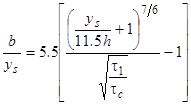Figure 1. Equation. Laursen's equation.

Where:

ys= Scour depth, ft.

h = Flow depth, ft.

b = Pier diameter, ft.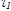= Grain bed shear, lb/ft2.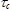= Critical shear at sediment threshold, lb/ft2.

In Laursen's equation, scour depends on the characteristics of the flow field (depth and bed shear), the structure (pier diameter), and the sediment (critical shear at sediment threshold). For the maximum potential scour depth, ys at=, the equation in figure 1 becomes figure 2.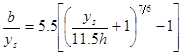Figure 2. Equation. Laursen's equation for potential maximum scour.

Since the scour depth, ys, is almost always much less than the flow depth, h, (11.5 ft), the equation in figure 2 is approximated by Ettema, et al., as shown in figure 3.(3)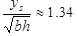Figure 3. Equation. Approximate maximum scour.

This result implies that the square root of the product of pier width and flow depth is the appropriate scaling length for pier scour depth.

### CSU EQUATION

The widely used CSU equation, which is described in HEC-18, resulted from a series of studies by Shen et al., Richardson and Davis, and Molinas. (See references 2 and 15–17.) Pier scour is estimated as shown in figure 4.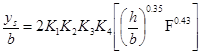Figure 4. Equation. CSU equation.

Where:

F = Froude number (F = V/(gh)^0.5).

K1 = Correction for pier shape (K1 = 1 for circular piers).

K2 = Correction for attack angle of approach flow (K2 = 1 for direct approach flow).

K3 = Correction for bed form (K3 = 1.1 for clear-water scour).

K4 = Correction for armoring (K4 = 1 for sand bed material).

For circular piers under clear-water scour conditions, the equation in figure 4 is rewritten as figure 5.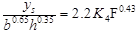Figure 5. Equation. CSU equation for clear-water scour at circular piers.

In this case, the implied length scaling for scour depth is b0.65h0.35. In addition, scour depth increases with Froude number and decreases with increasing armoring. Armoring is represented in K4 as a function of median grain size(D50), D95 sediment size where 95 percent of the sediment is finer by weight, and approach velocity (V).

The equations in figure 4 and figure 5 provide reasonable scour depths for many situations with narrow and intermediate piers.(3) Note that a pier is considered narrow when b/h < 0.71 and wide when b/h > 5.0. However, the use of the Froude number may not be physically representative of scour mechanisms if h is not very small. This is because the Froude number describes the ratio of inertial to gravitational forces on the fluid, while scour is a phenomenon of the interaction of water and sediment at the bed.

### MELVILLE-CHIEW EQUATION

Based on experiments at the University of Auckland in Auckland, New Zealand and Nanyang Technological University in Singapore, Melville and Chiew concluded that pier scour can be effectively estimated by considering piers as narrow, intermediate, or wide.(11) The maximum potential scour depth, ys, is scaled by different lengths, as shown in figure 6.(18)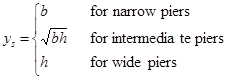Figure 6. Equation. Melville-Chiew equation.

Scaling for the intermediate pier scour in the equation in figure 6 is similar to that in the equation in figure 3. Melville and Chiew concluded that clear-water scour, ys, is almost proportional to V/Vc, with V representing approach flow velocity and Vc representing the critical approach velocity at the sediment movement threshold.(11) They further concluded that scour decreases as the sediment coarseness, D50/b, increases but that scour is independent of sediment size if D50/b ≤ 0.02 (fine sands).

### OLIVETO-HAGER EQUATION

Hager and Oliveto developed a scour relationship from studies at the Swiss Federal Institute of Technology in Zurich, Switzerland.(12,13,19) It was improved by Kothyari et al.(20) Hager and Oliveto stated that the flow-sediment interaction at the bed is best described by the densimetric particle Froude number, as shown in figure 7.(19)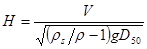Figure 7. Equation. Hager number.

Where:

H = Hager number (densimetric particle Froude number).

D50 = Median grain size, ft.

ρ = Water density, slug/ft3.

ρs = Sediment density, slug/ft3.

g = Gravitational acceleration, ft/s2.

This equation represents the effect of buoyancy, (ρs /ρ – 1)g, on the water-sediment interface. For brevity, H is referred to as the Hager number. Oliveto and Hager also developed the relationship shown in figure 8 for a cylindrical pier.(12,13)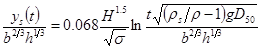Figure 8. Equation. Oliveto and Hager time-based scour.

Where:

ys(t)= Scour depth at time t, ft.
σ = Sediment non-uniformity (gradation coefficient).
t = Time, s.

This equation acknowledges the potential effect on scour depth by non-uniform sediments as reflected by the sediment non-uniformity parameter, σ. This parameter may also be referred to as a gradation coefficient. It is defined as the square root of the ratio of sediment size where 84 percent of the sediment is finer by weight (D84) to sediment size where 16 percent of the sediment is finer by weight (D16).

The equation in figure 8 does not indicate an equilibrium scour depth as time approaches infinity, but it is expected that for circular piers, equilibrium scour depth has the functional form shown in figure 9.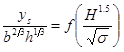Figure 9. Equation. Form for maximum scour.

The equation in figure 9 shows scour depth scaled to a combination of flow depth and pier width as is true for Laursen's equation and the CSU equation. In addition, the equation suggests that scour depth increases with the Hager number but decreases with sediment non-uniformity.

### SHEPPARD-MELVILLE EQUATION

The result of the evaluation of scour equations by Ettema et al. was to recommend that the CSU equation in HEC-18 be replaced with the Sheppard-Melville equation.(3) This equation is an integration of work by Sheppard and Miller and by Melville.(14,17) For clear-water scour, the Sheppard-Melville equation is described as shown in figure 10.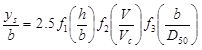Figure 10. Equation. Sheppard-Melville equation.

Vc may be estimated as described in HEC-18 as shown in figure 11.(2)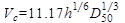Figure 11. Equation. Critical velocity.

In the relationship in figure 10, the function f1 represents the flow-structure interaction, the function f2 represents the flow-sediment interaction, and the function f3 represents the sediment-structure interaction. For the maximum potential scour depth, the equation in figure 10 is reduced by Ettema et al. to the equation in figure 12.(3)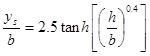Figure 12. Equation. Potential maximum scour derived from Sheppard-Melville.

When (h/b)0.4 is small (for shallow water or wide piers where h/b ≤ 0.3 ft), the equation is equivalent to figure 13.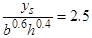Figure 13. Equation. Scour for shallow water or wide piers.

When (h/b)0.4 is large (for deep water or narrow piers h/b ≥ 10 ft), then the equation is equivalent to figure 14.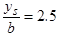Figure 14. Equation. Scour for deep water or narrow piers.

The Sheppard-Melville equation in figure 10 introduces the sediment-structure interaction through f3(D50/b), but since it is only based on D50, it does not capture the armoring effect for non-uniform bed materials.

### SUMMARY

Pier scour results from interactions between flow field, structure, and sediment characteristics. The objective is to capture these effects by the proper selection of independent variables.

The interaction between flow field and structure, as represented by pier width and flow depth, primarily dominates scour depth, as shown in figure 15 where the exponent, λ, is between zero and 1, inclusive.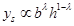Figure 15. Equation. Scour proportional to flow and structure parameters.

The interaction between flow field and sediment is a second factor governing scour depth. It is variously represented in terms of the ratio of grain bed shear to critical shear (/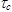), the ratio of approach velocity to critical velocity (V/Vc), or a function of the Hager number and sediment non-uniformity (H and σ). Since/and V/Vc can be converted to the Hager number through the Shield's diagram and a resistance equation described by Hager and Oliveto, the Hager number with sediment non-uniformity is used to represent the interaction between the flow field and sediment interaction, as shown in figure 16.(19)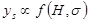Figure 16. Equation. Scour proportional to flow and sediment parameters.

The interaction between sediment and structure is a third factor governing scour depth. This factor is typically represented as the ratio of median grain size, D50, to pier width, b. Therefore, considering all three factors, scour depth, ys, may be described as shown in figure 17.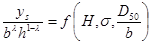Figure 17. Equation. Scour proportional to sediment and structure parameters.

The specific functional form for the equation in figure 17 is determined based on the understanding of scour mechanisms.

Federal Highway Administration | 1200 New Jersey Avenue, SE | Washington, DC 20590 | 202-366-4000
Turner-Fairbank Highway Research Center | 6300 Georgetown Pike | McLean, VA | 22101